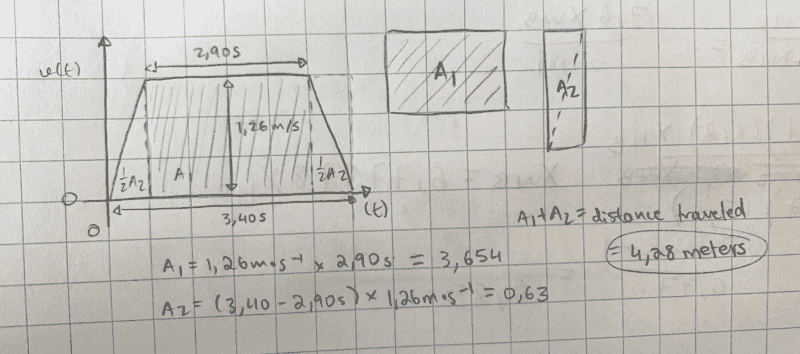# Intro Physics homework help - distance and velocity

• mncyapntsi

#### mncyapntsi

Homework Statement
An elevator going up from one floor to the next, where velocity is positive if it is upward. The acceleration at the start and the deceleration at the end are uniform and of the same magnitude. If the maximum speed of the elevator is 1.26 m/s and it takes a total of 3.40 s for the elevator to move between the floors, what is the distance between the floors if the time that the elevator maintains its top speed is 2.90 s?
Relevant Equations
v=d/t ; x = xo + vt
I have tried this problem over 4 times and keep getting the exact same answer, which is incorrect. Could someone please help by pointing out what I did wrong, or letting me know if I am actually on the completely wrong path?
Thanks!
Have a wonderful day :)Is the answer supposed to be ##3.97m##?

Is the answer supposed to be ##3.97m##?